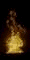Heat, Flow, Combustion, Problems, Analysis, Solutions, Help, Bastiaans### <!-- Begin var day=""; var month=""; var ampm=""; var ampmhour=""; var myweekday=""; var weekday=""; var year=""; mydate = new Date(); myday = mydate.getDay(); mymonth = mydate.getMonth(); myweekday= mydate.getDate(); weekday= myweekday; myyear= mydate.getYear(); year = myyear; myhours = mydate.getHours(); ampmhour = (myhours > 12) ? myhours - 12 : myhours; ampm = (myhours >= 12) ? ' PM' : ' AM'; mytime = mydate.getMinutes(); myminutes = ((mytime < 10) ? ':0' : ':') + mytime; if(myday == 0) day = " Sunday, "; else if(myday == 1) day = " Monday, "; else if(myday == 2) day = " Tuesday, "; else if(myday == 3) day = " Wednesday, "; else if(myday == 4) day = " Thursday, "; else if(myday == 5) day = " Friday, "; else if(myday == 6) day = " Saturday, "; if(myweekday == 1) weekday = "1st "; else if(myweekday == 21) weekday = "21st "; else if(myweekday == 2) weekday = "2nd "; else if(myweekday == 22) weekday = "22nd "; else if(myweekday == 23) weekday = "23rd "; else if(myweekday == 3) weekday = "3rd "; else if(myweekday == 31) weekday = "31st "; else weekday = myweekday + "th "; if(mymonth == 0) {month = "January";} else if(mymonth ==1) month = "February"; else if(mymonth ==2) month = "March"; else if(mymonth ==3) month = "April"; else if(mymonth ==4) month = "May"; else if(mymonth ==5) month = "June"; else if(mymonth ==6) month = "July"; else if(mymonth ==7) month = "August"; else if(mymonth ==8) month = "September"; else if(mymonth ==9) month = "October"; else if(mymonth ==10) month = "November"; else if(mymonth ==11) month = "December"; r = myyear - 100; if (myyear >= 1999) year = " " + myyear; else if(r < 10) year = " 200" + r; else year = " 20" + r; //--># Objectives

 The Combustion Institute was founded in 1954 to provide multiple ways for combustion scientists and engineers to communicate with each other and the rest of the technical world. On international level, for many years, this communication has been through the journals Combustion and Flame and The Proceedings of The Combustion Institute, as well as many conferences, particularly the biennial International Symposium on Combustion. The website of the international office of the Combustion Institute is located at Combustion Institute The Combustion Institute has many national sections, one of which is the Dutch Section. The Dutch section organises activities in the Netherlands or in collaboration with sections of other countries. In particular the Dutch Section finances the prize for the best posters at the annual Combura Symposium The current board of the Dutch Section has the following members: President: dr. ir. R.J.M. Bastiaans Secretary: Prof. dr. D.J.E.M. Roekaerts Treasurer: dr. Ir. J.B.W. Kok